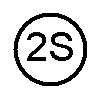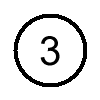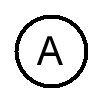For the 1858 half penny there are five die varieties consisting of five obverse dies paired with three reverse dies.

 1858Half Penny Obverse Reverse• Obverse 1:
• The spacing between the one and the eight is 0.90 mm.
• The spacing between the eight and the five is 0.69 mm.
• The spacing between the five and the eight is 0.40 mm.
• The eight and the five in the date have been repunched.
• Obverse 1S:
• Same as type 1 but striations are visible.
• Obverse 2S:
• The spacing between the one and the eight is 0.79 mm.
• The spacing between the eight and the five is 0.71 mm.
• The spacing between the five and the eight is 0.79 mm.
• The eight and the five in the date have been repunched.
• Striations are visible.
• Obverse 3:
• The spacing between the one and the eight is 0.79 mm.
• The spacing between the eight and the five is 0.89 mm.
• The spacing between the five and the eight is 0.75 mm.
• Obverse 4:
• The spacing between the one and the eight is 0.79 mm.
• The spacing between the eight and the five is 0.90 mm.
• The spacing between the five and the eight is 0.77 mm.
• The five has been repunched.

• Reverse A:
• There is a gap between the numerator and the bar in the fraction.
• Reverse B:
• The numerator touches the bar in the fraction.
• Reverse C:
• There is a significant die crack in the fraction.The spacings are measured between the digits.

Obverse Dies:  The DateSmall Spacing between the 8 and the 5
Repunched 8 and 5Small Spacing between the 8 and the 5Large Spacing between the 8 and the 5Large Spacing between the 8 and the 5
Repunched 5Reverse Dies:  1/26Gap between the numerator and the barNumerator touches the barSignificant die crackReverse Dies:  1/26Gap between the numerator and the barNumerator touches the barReverse Dies:  1/26Significant die crack